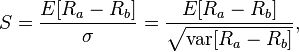# Bash script calculating sharpratios on Yahoo Finance dataSharpratio

For one of my trading projects I am looking to calculate something called sharpratio for pricing of an equity over time. This can be done in a spreadsheet, but this becomes a bit laborious as I want to do this for more than 1000 of data sets. So I set out to write a bash script that calculates the sharp ratio on any given price data set from Yahoo Finance. If you are interested in how I use this as part of a trading strategy. I will be posting there shortly on how I intend to use the below code as part of the trading strategy I use. More info on the theory of sharp ratio can be found here.

Here is what the code does:
It reads in a price data file that you can get from Yahoo Finance. The script will run through the file and will calculate the price difference for each trading day from adjusted close to adjusted close the other day. It will also calculate the absolute return made since the starting date of the data set. Then at the end, it will calculate the average of the daily differences, the standard deviation of these differences and finally the sharp ratio over the set of data.

```#!/bin/bash
# this script will read in a ohlc file from yahoo finance line by line
# putting every line in a list / array as an element
# and then do some basic calculations on the numbers
# we will calculate the absolute return, the avarage daily differences,
# the standard deviation of the daily differences and finally the sharp ratio

fname=\$1

do
filearray+=("\$line")
done &lt; \$fname
}

# initialize some variables we need later for calculations in the loop
absret=0
dailydifsq=0
sumofdailydifsq=0
stdev=0
# now let's loop through the array
# skip index zero because we use it as base: we cannot compare it to index -1 there is no.
for ((i=1; i &lt; \${#filearray[*]}; i++))
do
#echo  \$i
daybefore=`(echo \${filearray[\$i-1]}) | awk -F , '{print \$7}'`
today=`(echo \${filearray[\$i]}) | awk -F , '{print \$7}'`
dailydif=\$(echo "scale=4;((\$today-\$daybefore)/\$daybefore)*100"|bc)
echo "daily difference is \$dailydif %"
absret=\$(echo "scale=4;\$absret+\$dailydif"|bc) #sum of daily dif = abs return
echo "abs return sofar is \$absret"
dailydifsq=\$(echo "scale=4;\$dailydif*\$dailydif"|bc) # needed for calculating the stdev
sumofdailydifsq=\$(echo "scale=4;\$sumofdailydifsq+\$dailydifsq"|bc) # we wanna have the sum of all squared daily difs

done
avdd=\$(echo "scale=4;\$absret/\$i"|bc) #average dailydiff
stdev=\$(echo "scale=4;sqrt(\$sumofdailydifsq/\$i)"|bc) # here we use the sum of all daily difs, devide it by the count, and then take the square root.
sharpratio=\$(echo "scale=4;(\$avdd/\$stdev)*sqrt(252)"|bc)
#sharpratio is the avarage daily dif devided by the stdev and then multiplied by the sqrt of 252 for dauly pricing

echo "abs return has been \$absret%"
echo "av daily diff has been \$avdd%"
echo "stdev of daily diff has been \$stdev"
echo "the sharpratio has been \$sharpratio"
}